GET THE APP

Analyzing the Evolution of Biochemical Reaction System with a Com# Enzyme EngineeringOpen Access

Review Article - (2013) Volume 2, Issue 2

# Analyzing the Evolution of Biochemical Reaction System with a Complex Network Based Approach

Shu-Qiang Wang1* and Yan-Yan Shen2
1Department of Orthopaedics and Traumatology, The University of Hong Kong, Hong Kong, Hong Kong
2Department of Mechanical and Biomedical Engineering, City University of Hong Kong, Hong Kong, Hong Kong
*Corresponding Author: Shu-Qiang Wang, Department of Orthopaedics and Traumatology, The University of Hong Kong, Hong Kong Email:

## Abstract

Complex network theory has recently been used in System biology, but so far the resulting networks have only been analyzed statically. In this work, the biochemical reaction network (BRN) model is proposed based on the complex network theory and the dynamics of the network is analyzed on the molecular-scale. Given the initial ate and the evolution rules of the biochemical network, we demonstrated how the biochemical reaction network achieving homeostasis. The evolution of the biochemical reaction network is studied in perspective of average degree and edges. Comparing with the network features of random graphs, the network features from the proposed BRN model can reveal more biological sense.

Keywords: Evolution of BRN, Complex network, Network homeostasis, Dynamical analysis

#### Introduction

Network analysis has been applied widely, providing a unifying language to describe disparate systems ranging from social interactions to power grids. The notion of complex networks has become more and more popular for research in physics, engineering, biology and even social sciences [1-6]. A network can be viewed as a graph consisting of nodes connected by edges according to certain rules, in which the nodes and edges typically represent some physical, biological or social entities and their relationships, respectively [7,8]. Graph representation has been applied in many fields of scientific studies. For example, the Internet is a complex network of routers and computers linked by various physical or wireless links; fads and ideas spread on the social network, whose nodes are human beings and whose edges represent various social relationships; the World Wide Web is an enormous virtual network of Web pages connected by hyperlinks. These systems represent just a few of the many examples that have recently prompted the scientific community to investigate the mechanisms that determine the topology of complex networks [9,10].

Systems biology aims at investigating cellular networks by combining experiments, mathematical modeling and computer simulations. One fundamental prerequisite for the construction of cell models is the analysis of metabolic and signaling networks. The description of such systems comprises both the network structure and the reaction kinetics: only if both are known, stationary states, time courses and responses to parameter changes can be computed. Complex network theory has also been employed in molecular biology, but so far the resulting networks have only been analyzed statically [11-17]. In this work, we present the dynamics of biochemical reaction network on the molecular-scale. The rest of this paper is organized as follows. Section 2 gives the background knowledge of biochemical reaction and presents the problem formulation. In section 3, the Biochemical Reaction Model (BRN) is proposed and the algorithm for the biochemical reaction network is presented. Section 4 contains the simulation results for the biochemical reaction network in the initial state and equilibrium state, and the analytical comparisons between the BRN model and the proposed model. Finally, conclusions are drawn in Section 5.

#### Problem Statement

In this paper, we shall be concerned with the following general problem: N biochemical species or proteins are distributed randomly. Some species may have a higher probability to interact, while some other species may have a lower probability to interact. The interaction probability for any two biochemical species is determined by the distance between them, as well as their activities. The biochemical species will be re-arranged after their interaction. Given the original distribution of these biochemical species and the rules of evolution, what are the relationships among these biochemical species at any later time?

The concentration of species (protein) is an important index in biochemical reaction. In traditional literature, the concentration is defined as n/v. Here n is the amount of protein, which is always changing in biochemical reaction system, and V is the volume which is often fixed. In this model, we define the relative concentration of the protein i as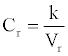(1)

Where, k is a constant. We call Vr the relative interaction volume of protein A and B. Vr is defined as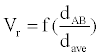(2)

Where d(A, B) is the distance between protein A and B and dave the average distance. It is easy to find that the relative volume is varying, which will induce variation of protein concentration.

We demonstrate the evolution of the biochemical reaction system via simulation. Each node here indicates one biochemical species or protein. Two nodes are connected when they start to interact.

#### Biochemical Reaction Network Model

In this section, we propose the biochemical reaction network (BRN) model based on the random graph theory. We study the dynamics of the biochemical reaction network by analyzing the evolution of the average degrees and edges. The system equation describing the relationships among these proteins is given by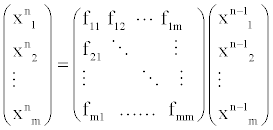(3)

Where, xin denotes protein i in the n state and fij denotes the transfer function between protein i and j.

The evolution of the biochemical reaction network is governed by the following rules:

(2) Calculate the interaction probability for any two biochemical species. The interaction probability can be expressed as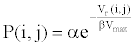(4)

Where, α, β are biochemical parameters determined by the activity of biochemical species.

We define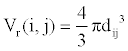(5)

And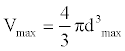(6)

Where, dij indicates the distance between node i and node j. (1<=i<=N, 1<=j<=N). dmax indicates the maximum distance between node i and the origin of coordinates.

(3) Compare the interaction probability p (i,j) with r. If p(i, j)>=r, node i and node j is connected .

(4) Break the connection between node i and node j and reset the location of node i and j.

(5) Repeat step 2-4 until the equilibrium state of biochemical interaction system is achieved.

#### Simulations and Analysis

Prior to studying the topological properties of the biochemical reaction network, we briefly define the following feature parameters:

• Average node degree, denoted by , is the average degree of all nodes in the network; here and represents the average operator.

• Average clustering factor, denoted by , is the average percentage of adjacently connected nodes.

ss

• Average path length, denoted by , is the average number of steps along the shortest paths for all possible pairs of as biological adaptation and calcium homeostasis in mammals . In this section, we demonstrate how the biochemical reaction network (BRN) achieving equilibrium state by studying the evolution of average degrees and edges.

The biochemical reaction in real-world is a stochastic process. A new series of random numbers is needed to simulate the real biochemical reaction network. Figure 1 and 2 show the evolutions of the average degrees and edges, respectively.

The equilibrium states shown in Figure 1 and 3 demonstrate the real homeostasis in biochemical system. In the real biochemical system, the average degree and the edges always vary on a small-scale even if the system achieves homeostasis.

From Figure 1 and 2, we can find that the average degrees and edges are not increasing monotonically. Figure 3 can gives us a deeper insight into the evolution of the node degrees before the system reaches the equilibrium state. Figure 3 shows that the probability of high degrees, such as k=50, becomes larger and larger as the cycling numbers increases, which is in conformity with the conclusion of Figure 1 and 2. And this is also in accordance with performance of the biochemical reaction system in real world: as homeostasis is closer and closer, the interaction frequency becomes higher and higher.

Figure 4 shows the degree distribution in the initial state, while Figure 5 shows the corresponding degree distribution in the equilibrium state. The parameters used in the simulation are α=0.15, β=0.25, N=100.

#### Comparison with the Original Random Graph

In this section, the comparisons between the original random graph model and the proposed BRN model are given. Basic network features, namely, degree distribution, total number of edges, average node degree, average clustering factor and average shortest path, are examined respectively.

Table 1 shows the basic network features of the original random graph and BRN, respectively. From Table 1, we can find that BRN has lager average degree, clustering coefficient and edges than those of the original random graph. However, when it comes to the average path length, Things reverses. This demonstrates the superiority of biological evolution. Figure 6 and 7 show the probability distribution of degrees for the original random graph and BRN, respectively.

 α=0.12  β=0.22 Original random graph BRN (Initial state) BRN (Homeostasis) Total number of edges 198 402 614 Average node degree 324 8.1 11.5 Average clustering factor 0.05604 0.083947 0.098671 Average path length Inf 2.6072 2.3841 α=0.2   β=0.3 Original random graph BRN (Initial state) BRN (Homeostasis) Total number of edges 452 586 831 Average node degree 8.42 11.32 16.53 Average clustering factor 0.0837 0.1295 0.1537 Average path length 2.3251 2.0594 1.7358 α=0.38   β=0.28 Original random graph BRN (Initial state) BRN (Homeostasis) Total number of edges 934 1451 1983 Average node degree 18.625 26.83 37.31 Average path length 1.6735 1.4831 1.2492

Table 1: Feature parameters of original random graph model and BRN model.

#### Conclusions

There are two recent developments in systems biology: first, biochemical networks can be easily extracted from databases; second, attempts are made to use this knowledge for large-scale or wholecell modeling without caring about individual reaction kinetics. To challenge these approaches, we attempted to extract information about biochemical network dynamics from the network structure, with very restricted knowledge about the individual reaction kinetics.

In this paper, The BRN model is proposed. The biochemical reaction system is studied using the random graph theory. The dynamics of the BRN is studied by analyzing the evolutions of some basic network features, such as average degrees and edges. The network features of BRN are demonstrated in both its initial state and equilibrium state. Furthermore, we compare the BRN with the original random graph by analyzing their network features. Our work provides a possible direction to study biochemical reaction system using complex network theory.

#### References

Citation: Wang SQ, Shen YY (2013) Analyzing the Evolution of Biochemical Reaction System with a Complex Network Based Approach. Enz Eng 2:113.

Copyright: © 2013 Wang SQ, et al. This is an open-access article distributed under the terms of the Creative Commons Attribution License, which permits unrestricted use, distribution, and reproduction in any medium, provided the original author and source are credited.
Top